# PostgreSQL Tutorial: MAX Function: Get Maximum Value in a Set

August 3, 2023

Summary: this tutorial shows you how to use the PostgreSQL `MAX()` function to get the maximum value of a set.

## Introduction to PostgreSQL MAX function

PostgreSQL `MAX` function is an aggregate function that returns the maximum value in a set of values. The `MAX `function is useful in many cases. For example, you can use the `MAX` function to find the employees who have the highest salary or to find the most expensive products, etc.

The syntax of the `MAX` function is as follows:

``````MAX(expression);
``````

You can use the `MAX` function not only in the `SELECT` clause but also in the `WHERE` and `HAVING` clauses. Let’s take a look at some examples of using the `MAX` function.

## PostgreSQL MAX function examples

Let’s examine the `payment` table in the sample database.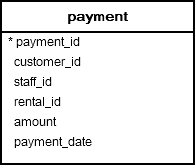The following query uses the `MAX()` function to find the highest amount paid by customers in the `payment` table:

``````SELECT MAX(amount)
FROM payment;
``````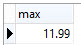### PostgreSQL MAX function in subquery

To get other information together with the highest payment, you use a subquery as follows:

``````SELECT * FROM payment
WHERE amount = (
SELECT MAX (amount)
FROM payment
);
``````

First, the subquery uses the `MAX()` function to return the highest payment and then the outer query selects all rows whose amounts are equal the highest payment returned from the subquery.

The output of the query is as follows: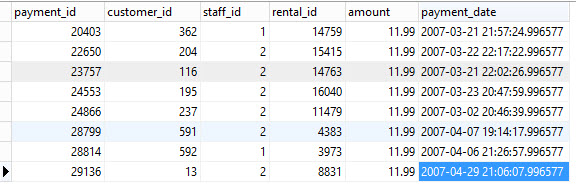The following diagram illustrates the steps that PostgreSQL performs the query:### PostgreSQL MAX function with GROUP BY clause

You can combine the `MAX `function with the `GROUP BY` clause to get the maximum value for each group. For example, the following query gets the highest payment paid by each customer.

``````SELECT
customer_id,
MAX (amount)
FROM
payment
GROUP BY
customer_id;
``````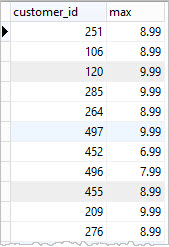### PostgreSQL MAX function with HAVING clause

If you use the `MAX()` function in a `HAVING` clause, you can apply a filter for a group.

For example, the following query selects only the highest payment paid by each customer and the payments are greater than `8.99`.

``````SELECT
customer_id,
MAX (amount)
FROM
payment
GROUP BY
customer_id
HAVING MAX(amount) > 8.99
``````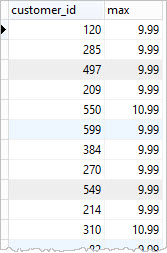## Finding the largest values from two or more columns

First, create a new table named `ranks` that consists of four columns: the first column stores user id and the other three columns to store ranks from 1 to 3.

``````DROP TABLE IF EXISTS ranks;
CREATE TABLE ranks (
user_id INT PRIMARY KEY,
rank_1 INT NOT NULL,
rank_2 INT NOT NULL,
rank_3 INT NOT NULL
);
``````

Second, insert sample data into the `ranks` table as follows:

``````INSERT INTO ranks
VALUES
(1, 6, 3, 5),
(2, 2, 8, 5),
(3, 5, 9, 8);
``````

How do you get the largest rank per user as shown in the screenshot below: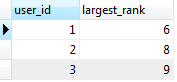To achieve this, you use `GREATEST()` function instead of `MAX` function. The `GREATEST` function returns the greatest value from a list of values.

``````SELECT
user_id,
GREATEST (rank_1, rank_2, rank_3) AS largest_rank
FROM
ranks;
``````

In this tutorial, you have learned how to use the PostgreSQL `MAX` function to find the maximum value of a set.